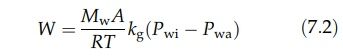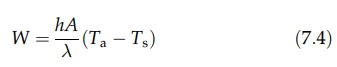# Evaporation of Water into an Airstream

| Home | | Pharmaceutical Technology |

## Chapter: Pharmaceutical Engineering: Drying

The evaporation of moisture into a warm airstream, the latter providing the latent heat of evaporation, is a common drying mechanism, although it is not easily adapted to the recovery of the liquid.

EVAPORATION OF WATER INTO AN AIRSTREAM

The evaporation of moisture into a warm airstream, the latter providing the latent heat of evaporation, is a common drying mechanism, although it is not easily adapted to the recovery of the liquid. We will consider first evaporation from a liquid surface, which, with the passage of air, falls to the wet bulb temperature corresponding to the temperature and humidity of the air, as described in chapter 6. The rate at which water vapor is transferred from the saturated layer at the surface to the drying stream is described by equation (4.5) in chapter 4 as:where Pwi is the partial pressure of the water vapor at the surface and Pwa is the partial pressure of water vapor in the air. kg is a mass transfer coefficient, and N is the number of moles of vapor transferred from unit area in unit time. Rewriting this in terms of the total mass, W, transferred in unit time from the entire drying surface, A,where Mw is the molecular weight of water vapor, R is the gas constant, and T is the absolute temperature.

The mass transfer coefficient, kg, will itself be a function of the temperature, the air velocity, and its angle of incidence. A high velocity or angle of incidence diminishes the thickness of the stationary air layer in contact with the liquid surface and, therefore, lowers the diffusional resistance.

The rate of evaporation may also be expressed in terms of the heat transferred across the laminar film from the drying gases to the surface. This is described by equation (3.7) in chapter 3 as:

Q = hA(Ta - Ts)                                (7:3)

where Q is the rate of heat transfer, A is the area of the surface, Ta and Ts are the temperatures of the drying air and the surface, respectively, and h is the heat transfer coefficient. The latter is also a function of air velocity and angle of impingement. If the latent heat of evaporation is λ, this affords a mass transfer rate, W, which is given byEquilibrium drying conditions are represented by the equality of equa-tions (7.2) and (7.4). When these conditions pertain to drying, the surface temperature, Ts, which is the wet bulb temperature, is normally much lower than the temperature of the drying gases. This is of great importance in the drying of thermolabile materials.

If solids are present in the surface, the rate of evaporation will be modified, the overall effect depending on the structure of the solids and the moisture content.